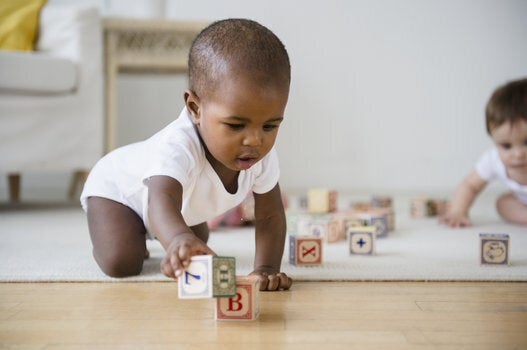# Name Numerology: What It Is And What Your Baby's Name Means

Numerologists believe the number value of a person’s name directly influences their personality, destiny and soul.Closeup photo of five month old baby boy (Asian/Filipino) on his tummy staring at the camera. He has a heart shaped mouth.

Your baby’s name can have meanings in different languages, but did you know it could have a numerical meaning as well?

People who study numbers, known as numerologists, believe the number value of a person’s name directly influences their personality and destiny.

They also believe that analyzing this number gives insight into what can help or hinder a person from having a successful life, similar to astrological signs.

How to calculate

So how do you figure out your baby’s name numerology?

The method is simple. In numerology, each letter is assigned a number from one to nine as follows:

1 = A, J, S

2 = B, K, T

3 = C, L, U

4 = D, M, V

5 = E, N, W

6 = F, O, X

7 = G, P, Y

8 = H, Q, Z

9 = I, R

To calculate a name’s value, add each number for the letters in the name. If the total number is a double-digit, simply add those two numbers together to get a single digit. (For example, if your name number is 21, the numerical value is three).

The only exception is if your name number is either 11 or 22. These numbers are not added together and are known as “master numbers” that have their own unique meaning.

Here are some examples:

Sofia, the most popular baby name in the world, gives us the equation 1+6+6+9+1, which equals 23. Two and three are then added together to give Sofia the numerical value of five.

Liam, one of Canada’s top baby names of 2015, gives us the equation 3+9+1+4, which equals 17. One and seven added together then give Liam the numerical value of eight.

And finally, Wes, which gives the equation 5+5+1, has the numerical value of 11. The numbers one and one are not added together because 11 is a master number.

Using this method of calculation, you can determine your baby’s destiny, personality and soul number. These calculations are done in different ways and reveal various insights.

Destiny number

This is the actual numerical value of your name. It is calculated using the letters of your first and last name and describes your overall character. It can also reveal potential strengths and weaknesses instilled in you at birth.

Personality number

This is calculated using the consonants in your first name only. It reveals your specific personality and how people perceive you.

Soul number

This number reveals your heart’s desire or soul yearning. It is calculated using the vowels in your first and last name. This number also represents what guides your inner self and reveals your private inner cravings.

Now that you know how to calculate the numerical value of your baby’s name, here is the meaning behind those numbers: